# Expressions and Macros in Julia

• Last Updated : 25 Aug, 2020

Metaprogramming is a way to form a well-built code. If this technique is used precisely then it can result in more condensed and readable code. Julia is a homoiconic language, it means that Julia can personify its own code as the data structure for the language itself. It is possible for the program to reconstruct its own code without any extra building steps. Another property of Julia, of being a metaprogramming language is the caliber of the running program to dynamically come across the properties of itself. Expressions and Macros are a vital part of metaprogramming in Julia.

### Expressions in Julia

Expressions are exceptional type objects in Julia language. A Julia code appears to be a syntax tree that is extended from Julia data structures of type Expr. This makes it simple to set up and undergo changes in Julia code from within Julia without deducing the original text.

Any chunk of code that has not been evaluated can be said to be an expression. There is a certain function that is used to evaluate expressions.

For instance,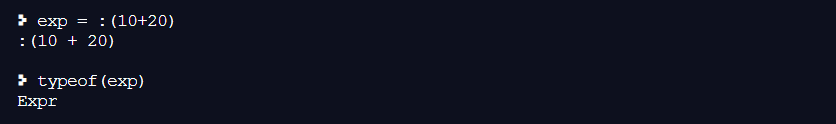The above piece of code just formed an object ‘Expr’ and it will remain as it is unless any function will take action towards that object, like eval(),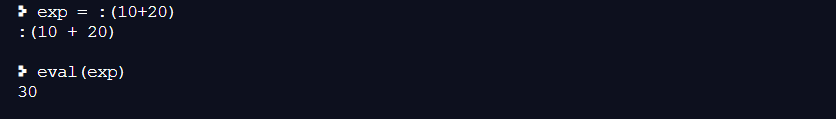This all seems to be a little complicated as you just need to add two numbers only. The reason behind this complex expression is that it unveils new robust capabilities to Julia programmers.

### Creation of Expressions

There are various ways to create expressions in Julia, some of them are listed below:

#### using Expr() Function

Julia provides a pre-defined function Expr() that can be used to create expressions of user’s choice.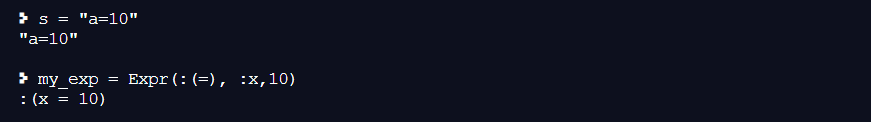Here, my_exp = Expr(:(=), :x, 10) is the prefix notation of the syntax, the first argument is head and the remaining are arguments of the expression. The head determines the operation to be performed, here the value 10 is assigned to x.

#### Quoting

The basic syntax of creating expression with quoting is colon(🙂 followed by parentheses () around the single statement of the code i.e. :()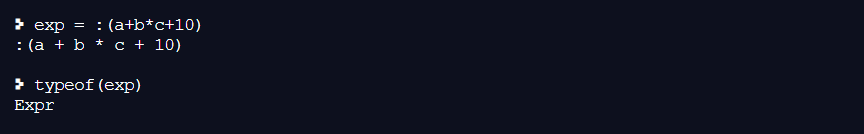You can construct this expression a*b+c+10 in various other ways like using Meta.parse and Expr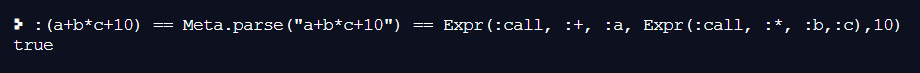#### using quote…end

This enables creating multi-line expressions.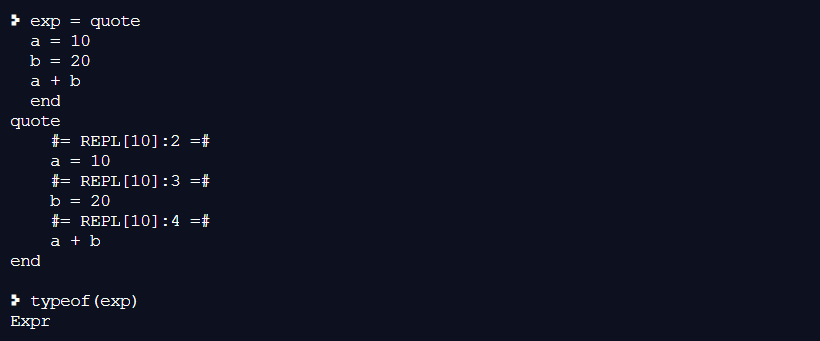#### Interpolation

Julia allows literals to get interpolated in expression i.e it allows values to get inserted into an expression.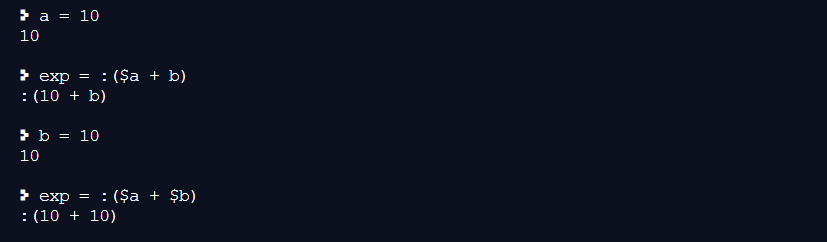Example of a tuple interpolated as an expression,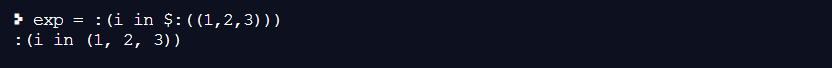Note: If the expression is unquoted, it will show compile-time error: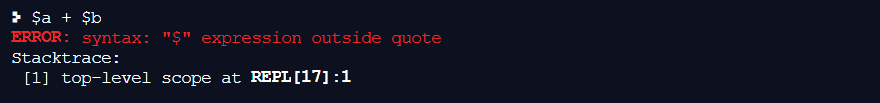\$ allows only a single expression to get interpolated, in case you want to insert multiple expression, use \$(exps…), where exps is an array of expressions.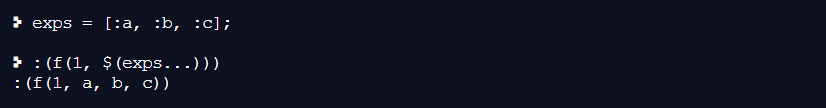#### Evaluating Expressions

Expressions can be evaluated using pre-defined eval() method.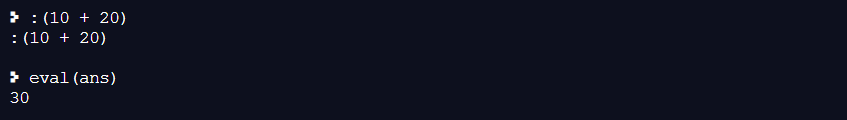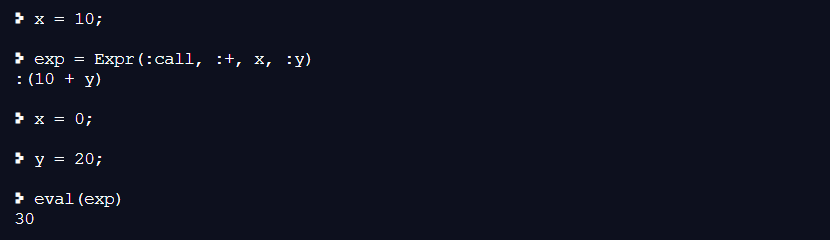In the above example, the value of a which is at the construction time of expression is treated as an intermediate value in the expression, therefore a=0 doesn’t matter. Here, the value of a is treated as 30.

#### Functions on Expressions

One extremely useful feature of Julia is the capability to generate and manipulate Julia code within Julia itself. We have already seen one example of a function returning Expr objects: the parse function, which takes a string of Julia code and returns the corresponding Expr. Expr objects can act as an argument for the functions too and the function returns another Expr. Here is an example,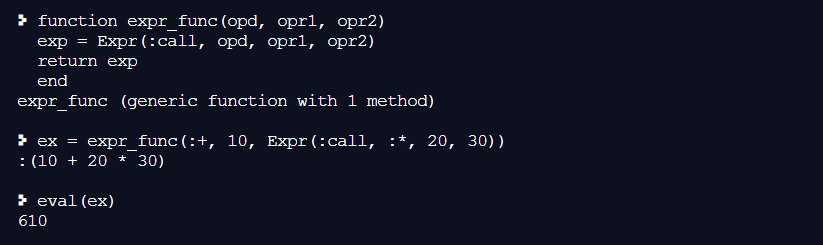### Macros in Julia

Now that you know, how to handle expressions in Julia, now there is the step to modify them and this is done using a macro. Macro is one of the ways to evaluate the input expression and gives the resultant output expression. The process in the Julia language works as follows: it first parses and evaluates the macro, and the processed code produced by the macro is eventually evaluated like an ordinary expression. Macro can be referred to as a code of code. Here is the syntax below:

```macro e(x)
if typeof(x) == Expr
println(x.args)
end
return x
end

```

Macros are run using the character ‘@’ as a prefix,

```@name exp1 exp2 ...
@name(exp1, exp2, ...)

```

These are two styles of writing and should not be mixed.

```julia> @e 10
10

```

On passing a number ’10’ it returns the number itself because number aren’t expressions. Here are some more examples of macro.

Example 1: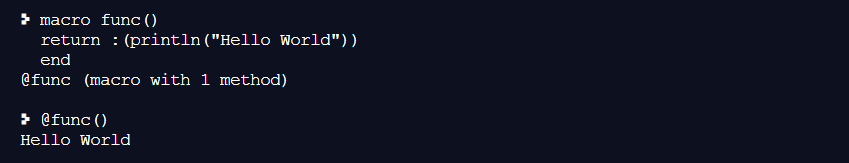Example 2: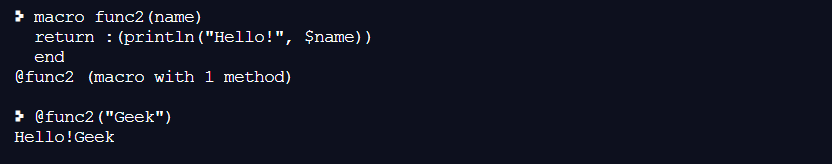There are certain ways to know the argument of the macro. This can be done using show() function within the macro body: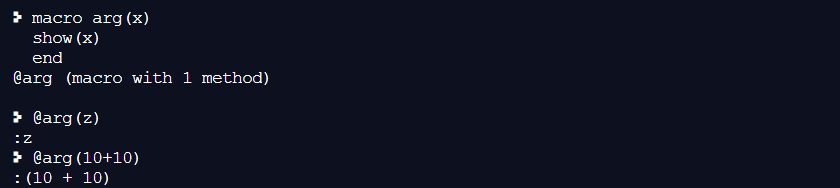Let’s look at @asset macro, below is the example: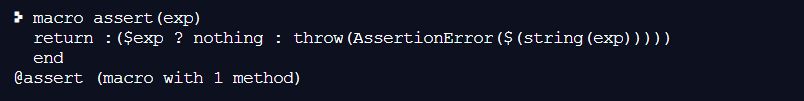It can be used as follows: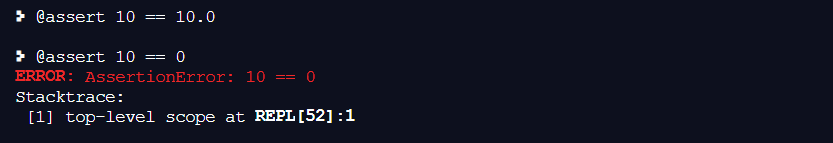The above code is actually like:

```10 == 10.0 ? nothing : throw(AssertionError("10 == 1.0"))
10 == 0 ? nothing : throw(AssertionError("10 == 0"))
```

This entire expression is placed into the syntax tree where the @assert macro call occurs and then at the time of execution if the test expression evaluates to true, then nothing is returned, whereas if the test is false, an error is raised indicating the asserted expression that was false.

Let’s look at another macro @eval. You must be thinking of eval() but these are different in some way. eval() is a function and @eval is a macro. You are familiar with the eval() function, it first expands and evaluates the expression, but @eval does not do the same. It does not expand the expression and if you want to evaluate the expression you can use the process of interpolation. This can be done as follows: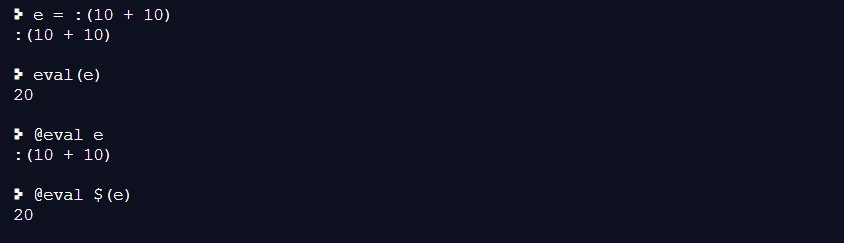Basically,

```@eval \$(ex) == eval(ex)

```

The above chunk of code will return true.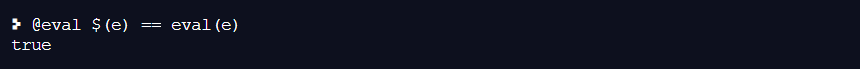My Personal Notes arrow_drop_up